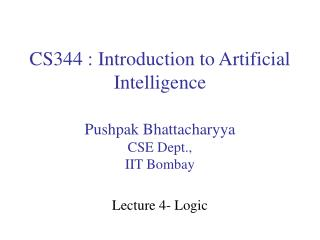Download PresentationCS344 : Introduction to Artificial Intelligence# CS344 : Introduction to Artificial Intelligence - PowerPoint PPT Presentation

Download Presentation##### CS344 : Introduction to Artificial Intelligence

Download Policy: Content on the Website is provided to you AS IS for your information and personal use and may not be sold / licensed / shared on other websites without getting consent from its author. While downloading, if for some reason you are not able to download a presentation, the publisher may have deleted the file from their server.

- - - - - - - - - - - - - - - - - - - - - - - - - - - E N D - - - - - - - - - - - - - - - - - - - - - - - - - - -
##### Presentation Transcript

1. CS344 : Introduction to Artificial Intelligence Pushpak BhattacharyyaCSE Dept., IIT Bombay Lecture 4- Logic

2. Logic and inferencing Vision NLP • Search • Reasoning • Learning • Knowledge Expert Systems Robotics Planning Obtaining implication of given facts and rules -- Hallmark of intelligence

3. Inferencing through • Deduction (General to specific) • Induction (Specific to General) • Abduction (Conclusion to hypothesis in absence of any other evidence to contrary) Deduction Given: All men are mortal (rule) Shakespeare is a man (fact) To prove: Shakespeare is mortal (inference) Induction Given: Shakespeare is mortal Newton is mortal (Observation) Dijkstra is mortal To prove: All men are mortal (Generalization)

4. If there is rain, then there will be no picnic Fact1: There was rain Conclude: There was no picnic Deduction Fact2: There was no picnic Conclude: There was no rain (?) Induction and abduction are fallible forms of reasoning. Their conclusions are susceptible to retraction Two systems of logic 1) Propositional calculus 2) Predicate calculus

5. Propositions • Stand for facts/assertions • Declarative statements • As opposed to interrogative statements (questions) or imperative statements (request, order) Operators => and ¬ form a minimal set (can express other operations) - Prove it. Tautologies are formulae whose truth value is always T, whatever the assignment is

6. Model In propositional calculus any formula with n propositions has 2n models (assignments) - Tautologies evaluate to T in all models. Examples: 1) 2) • e Morgan with AND

7. Semantic Tree/Tableau method of proving tautology Start with the negation of the formula - α - formula α-formula β-formula - β - formula α-formula - α - formula

8. Example 2: X (α - formula) (α - formulae) α-formula (β - formulae) B C B C Contradictions in all paths

9. Exercise: Prove the backward implication in the previous example

10. Formal Systems • Rule governed • Strict description of structure and rule application • Constituents • Symbols • Well formed formulae • Inference rules • Assignment of semantics • Notion of proof • Notion of soundness, completeness, consistency, decidability etc.

11. Hilbert's formalization of propositional calculus 1. Elements are propositions : Capital letters 2. Operator is only one :  (called implies) 3. Special symbolF(called 'false') 4. Two other symbols : '(' and ')' 5. Well formed formula is constructed according to the grammar WFF P|F|WFFWFF 6. Inference rule : only one Given AB and A write B known asMODUS PONENS

12. 7. Axioms : Starting structures A1: A2: A3 This formal system defines the propositional calculus

13. Notion of proof 1. Sequence of well formed formulae 2. Start with a set of hypotheses 3. The expression to be proved should be the last line in the sequence 4. Each intermediate expression is either one of the hypotheses or one of the axioms or the result of modus ponens 5. An expression which is proved only from the axioms and inference rules is called a THEOREM within the system

14. Example of proof From P and and prove R H1: P H2: H3: i) P H1 ii) H2 iii) Q MP, (i), (ii) iv) H3 v) R MP, (iii), (iv)

15. Prove that is a THEOREM i) A1 : P for A and B ii) A1: P for A and for B iii) A2: with P for A, for B and P for C iv) MP, (ii), (iii) v) MP, (i), (iv)

16. Formalization of propositional logic (review) Axioms : A1 A2 A3 Inference rule: Given and A, write B A Proof is: A sequence of i) Hypotheses ii) Axioms iii) Results of MP A Theorem is an Expression proved from axioms and inference rules

17. Example: To prove i) A1 : P for A and B ii) A1: P for A and for B iii) A2: with P for A, for B and P for C iv) MP, (ii), (iii) v) MP, (i), (iv)

18. Shorthand 1. is written as and called 'NOTP' 2. is written as and called 'PORQ’ 3. is written as and called 'P AND Q' Exercise: (Challenge) - Prove that

19. A very useful theorem (Actually a meta theorem, called deduction theorem) Statement If A1, A2, A3 ............. An├ B then A1, A2, A3, ...............An-1├ ├ is read as 'derives' Given A1 A2 A3 . . . . An B A1 A2 A3 . . . . An-1 Picture 1 Picture 2

20. Use of Deduction Theorem Prove i.e., ├ F(M.P) A├(D.T) ├(D.T) Very difficult to prove from first principles, i.e., using axioms and inference rules only

21. Prove i.e. ├ F ├ (D.T) ├ Q (M.P with A3) P├ ├